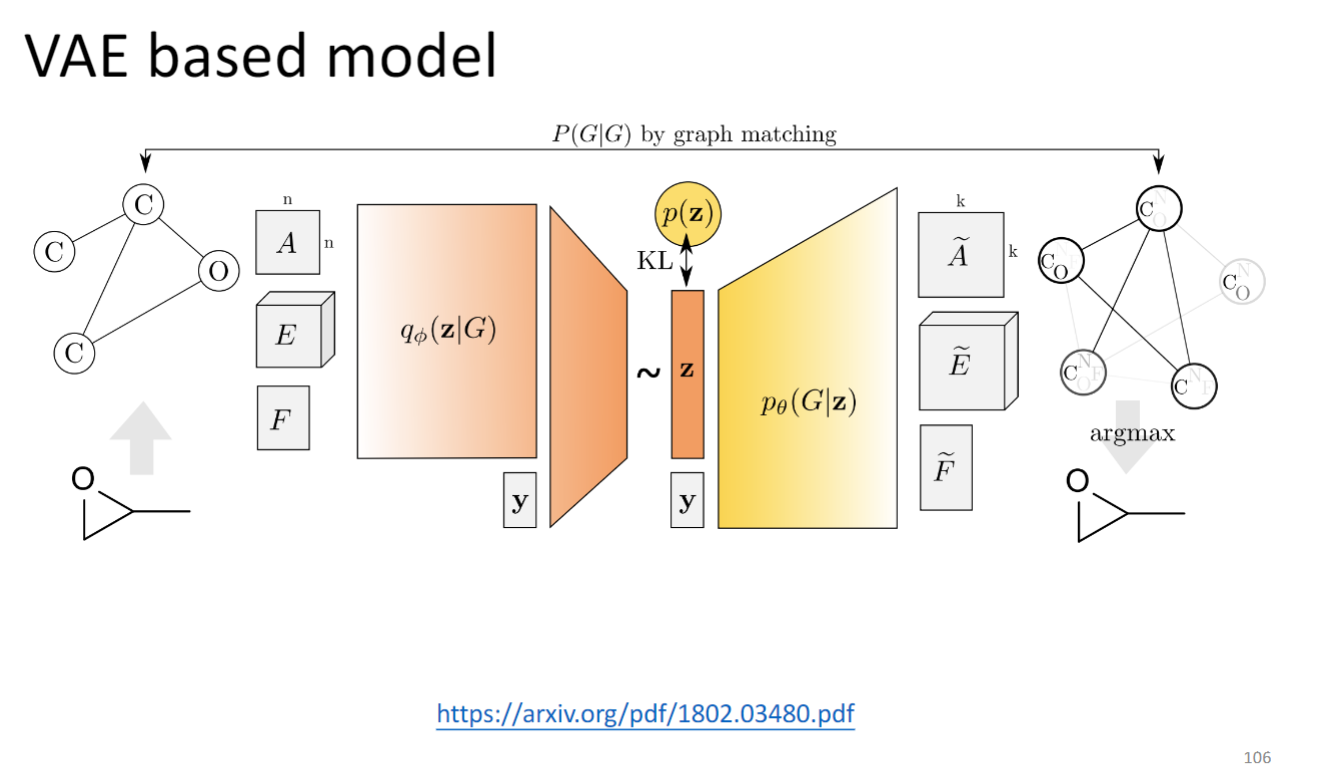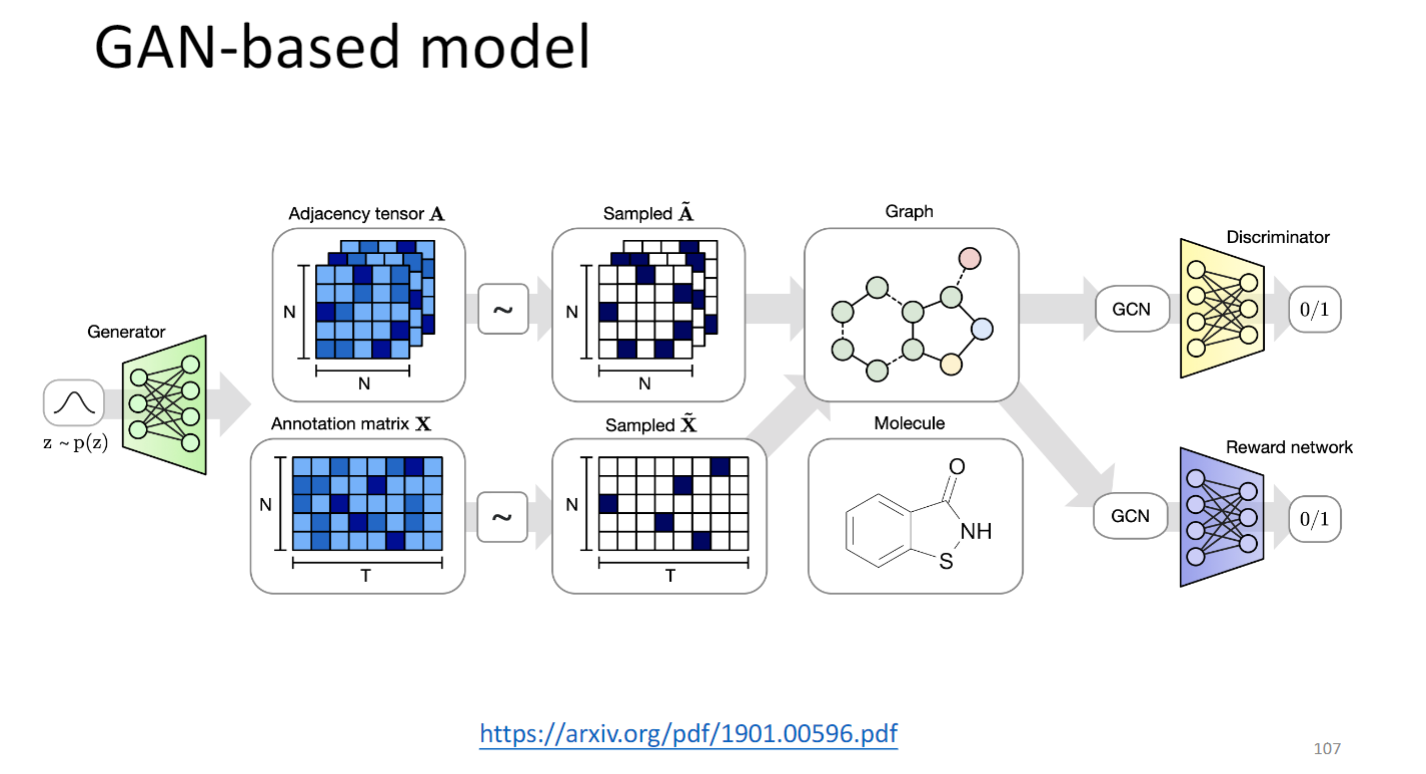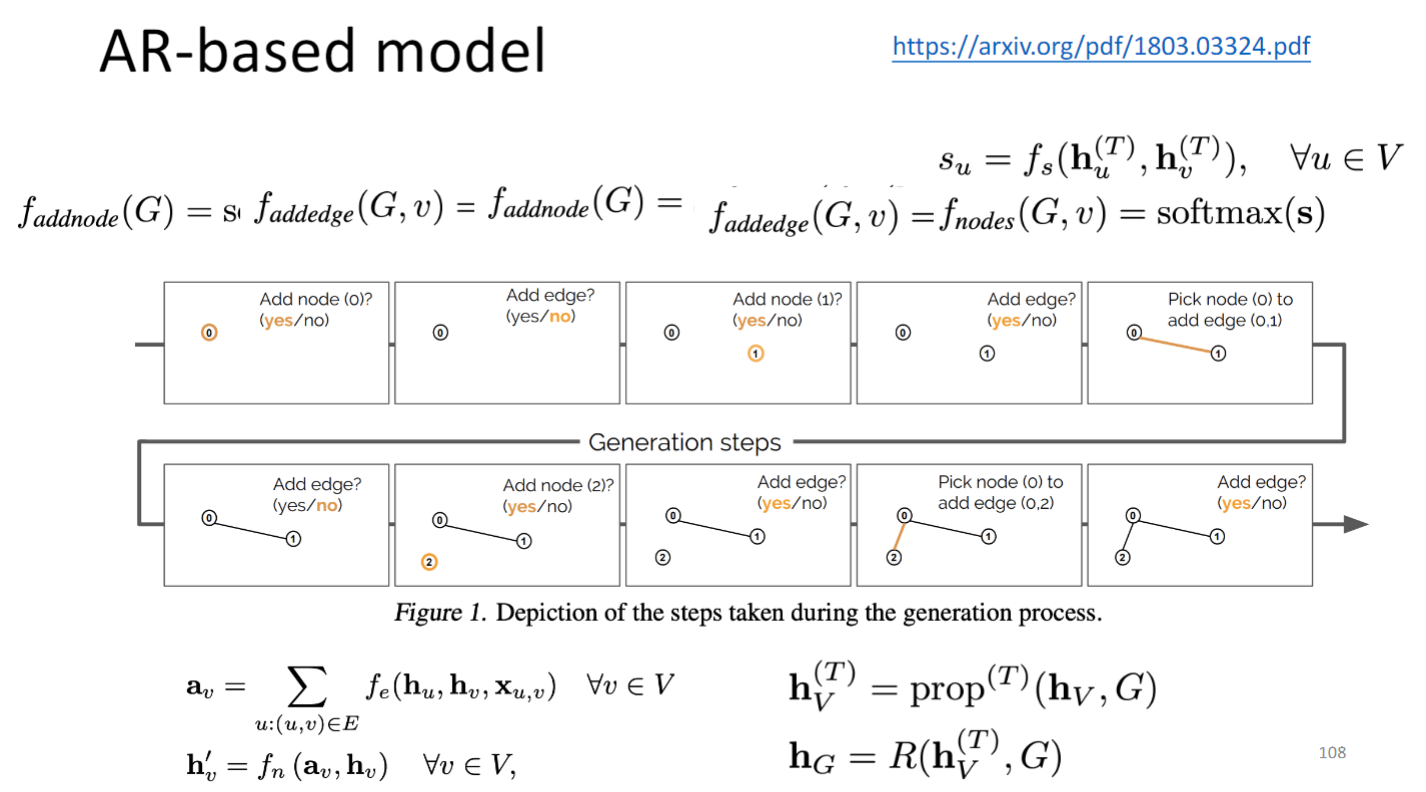# Graph Neural Network

## Introduction

### 定义

GNN 图神经网络 就是把图作为神经网络的输入，识别图结构，提取图信息，或生成特定结构的图的神经网络模型。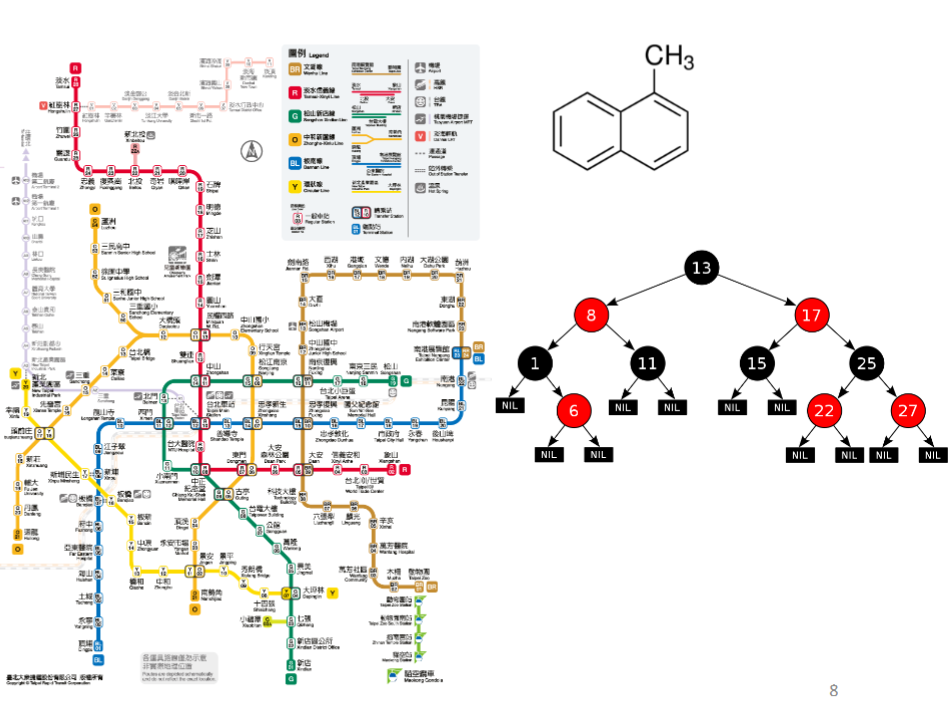### 训练GNN将遇到的问题

convolution？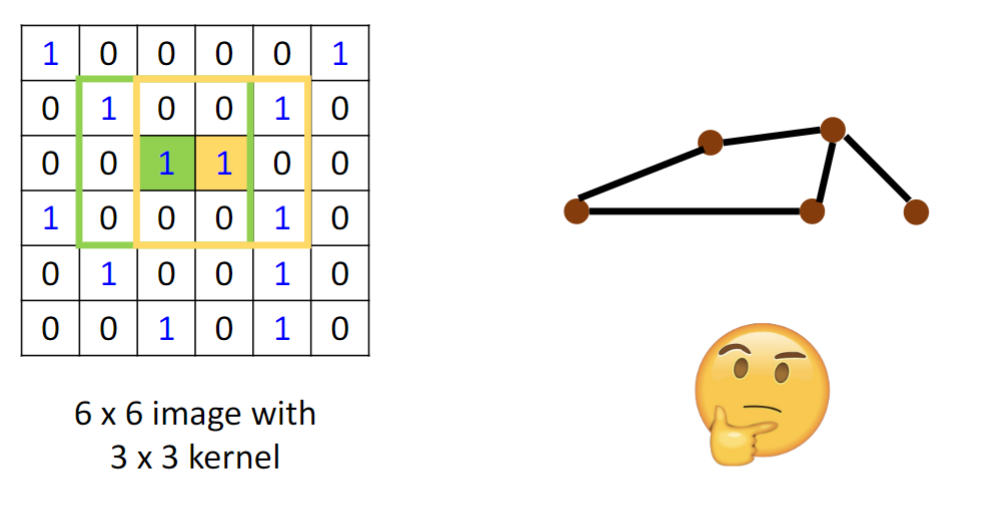• Solution 1: Generalize the concept of convolution (corelation) to graph >> Spatial-based convolution
• Solution 2: Back to the definition of convolution in signal processing >> Spectral-based convolution• Semi-supervised node classification
• Regression
• Graph classification
• Graph representation learning

Common dataset

• CORA: citation network. 2.7k nodes and 5. 4k links
• TU-MUTAG: 188 molecules with 18 nodes on average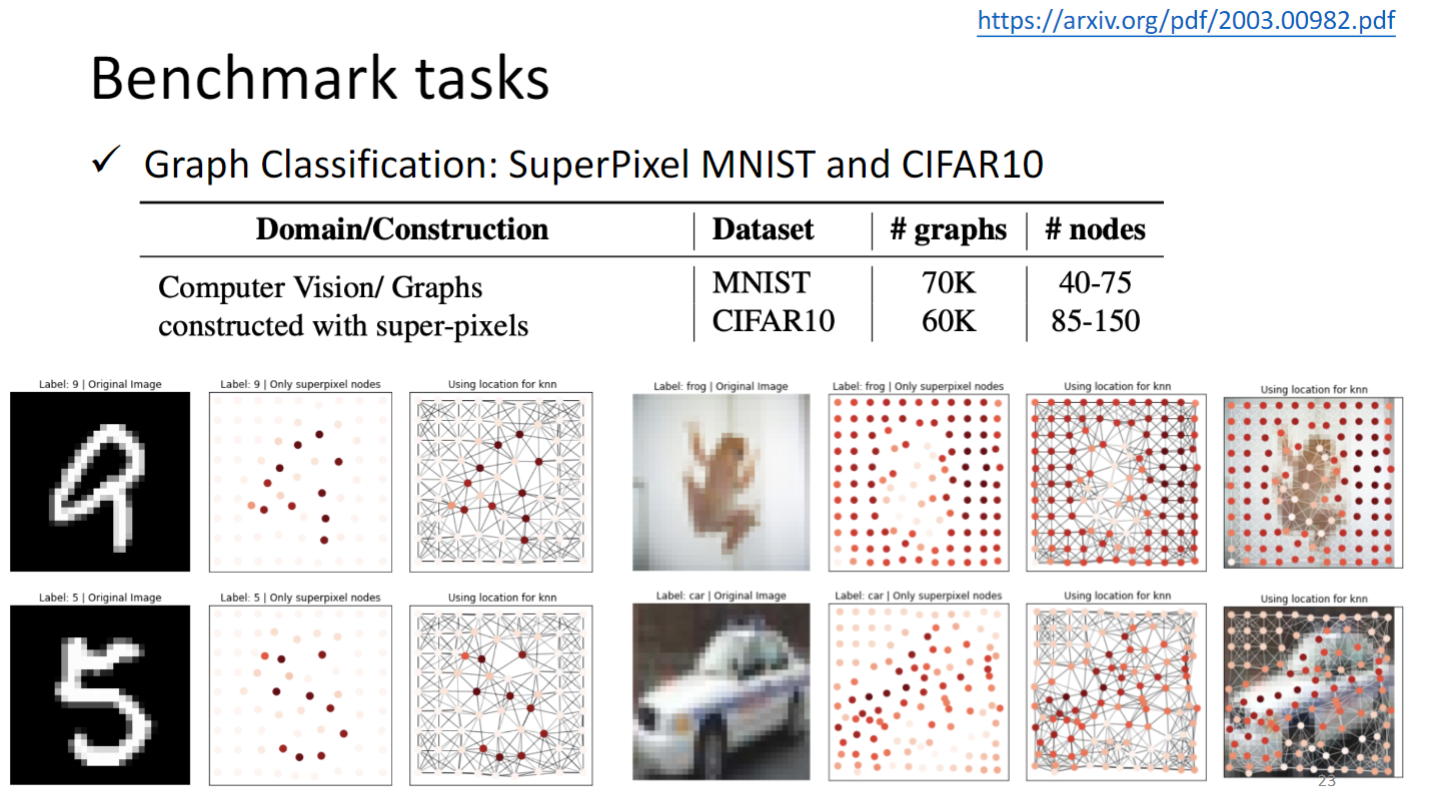ZINC 是通过分子graph 计算分子溶解度，属于回归任务

Stochastic Block Model dataset 是给出一个pattern，model要是别这个pattern是否出现在一个graph中TSP 路径规划问题，就不用赘述了.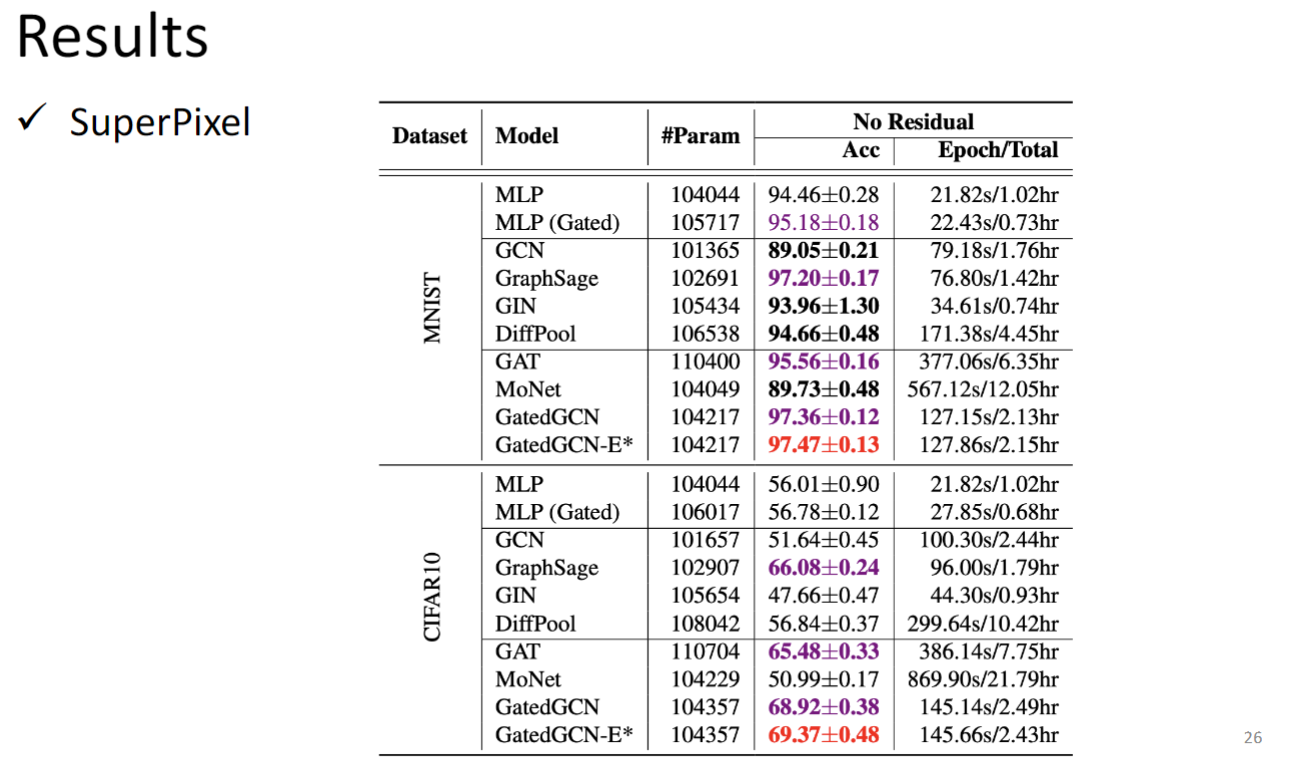## Spatial-based GNN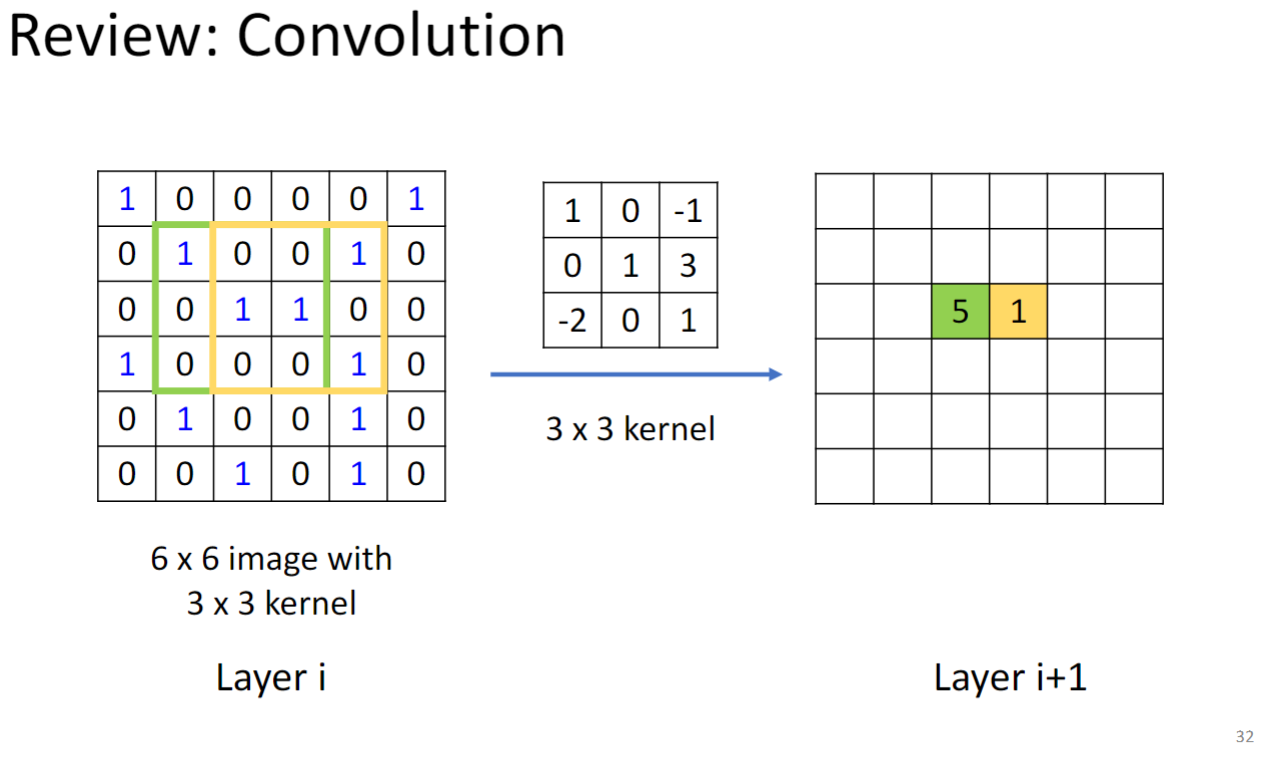CNN在layer i上通过kernel计算feature得到layer i+1层的feature，类比到Graph上：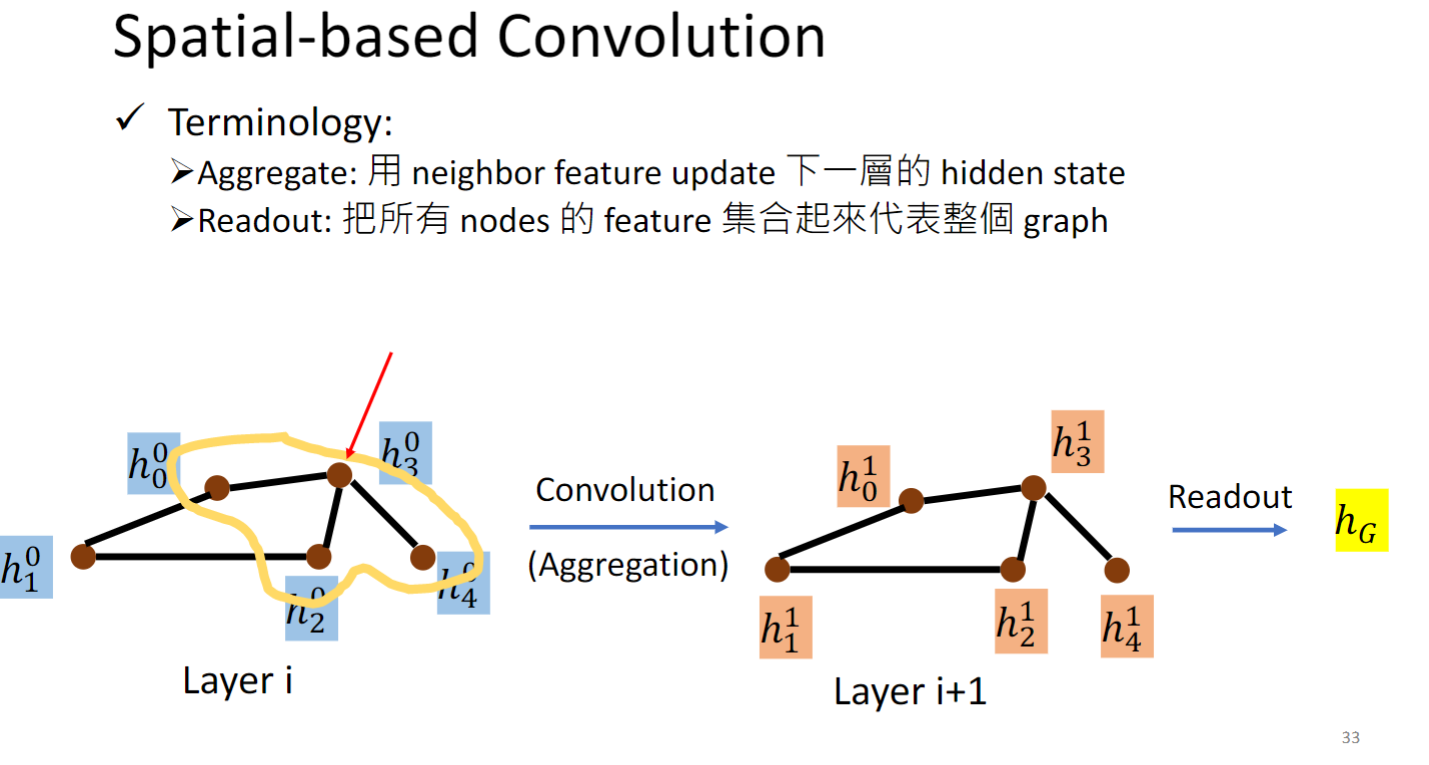$h_3^0$ 为例，0表示第0 layer，3表示第3个node，它的邻居是黄色圈起来的三个几点，我们就用这三个邻居的hidden feature 来算出下一层的hidden layer，这一招叫做Aggregation.

### NN4G(Neural Networks for Graph)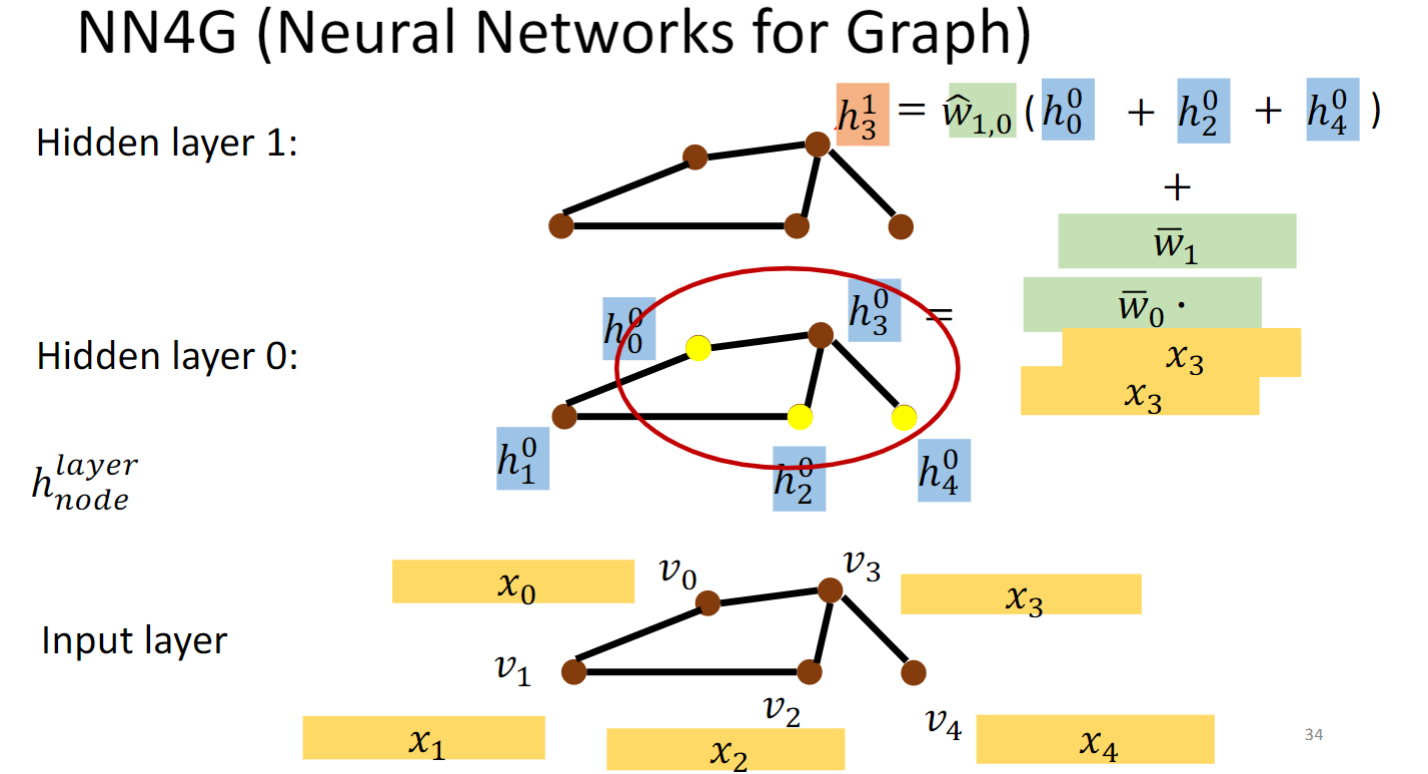NN4G这个模型的做法如上图所示，每个节点v都用一个特征向量x表示，对每个节点做embedding，即乘一个矩阵w，得到h，然后从一个hidden layer到下一个hidden layer做Aggregation，具体做法就是将一个节点的邻居的h加起来乘上一个w，就得到这个节点在下一个hidden layer的h.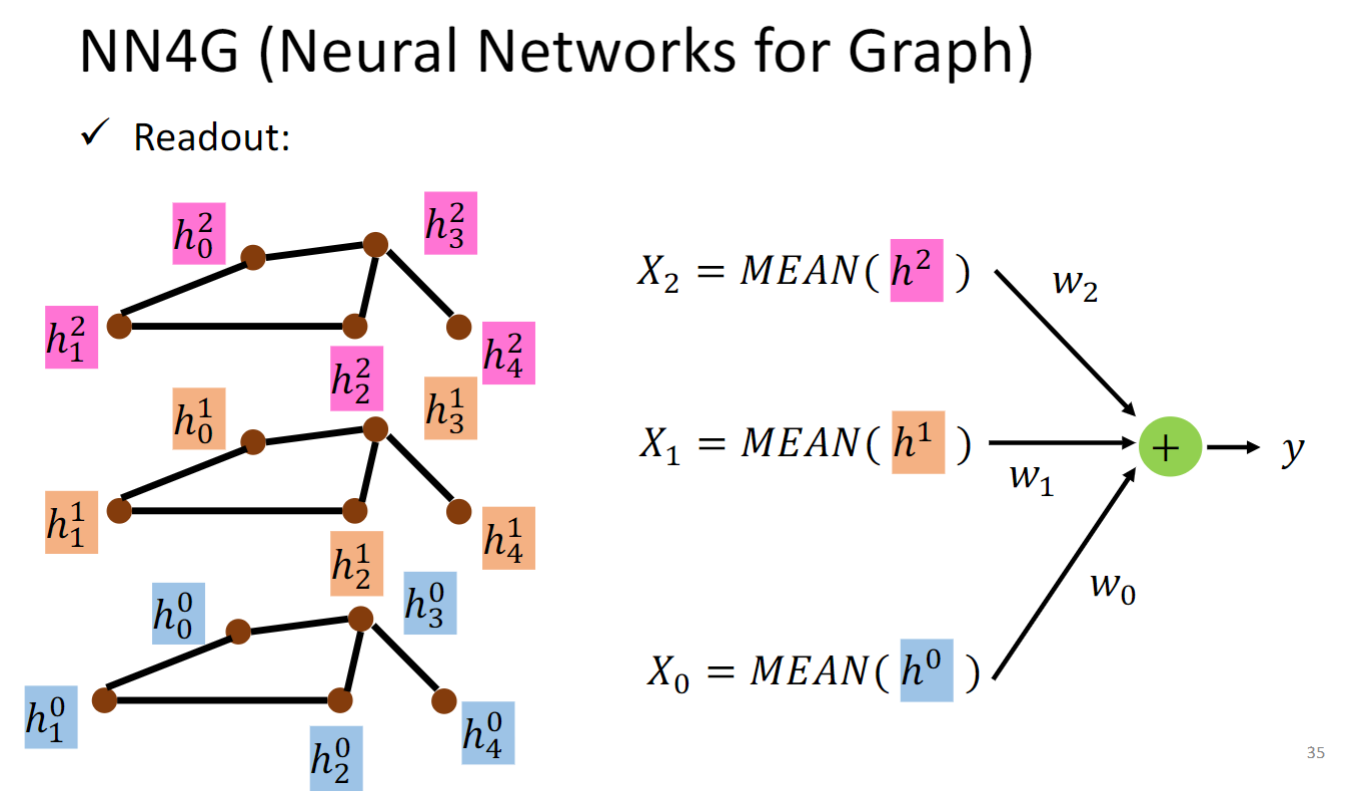### DCNN(Diffusion-Convolution Neural Network)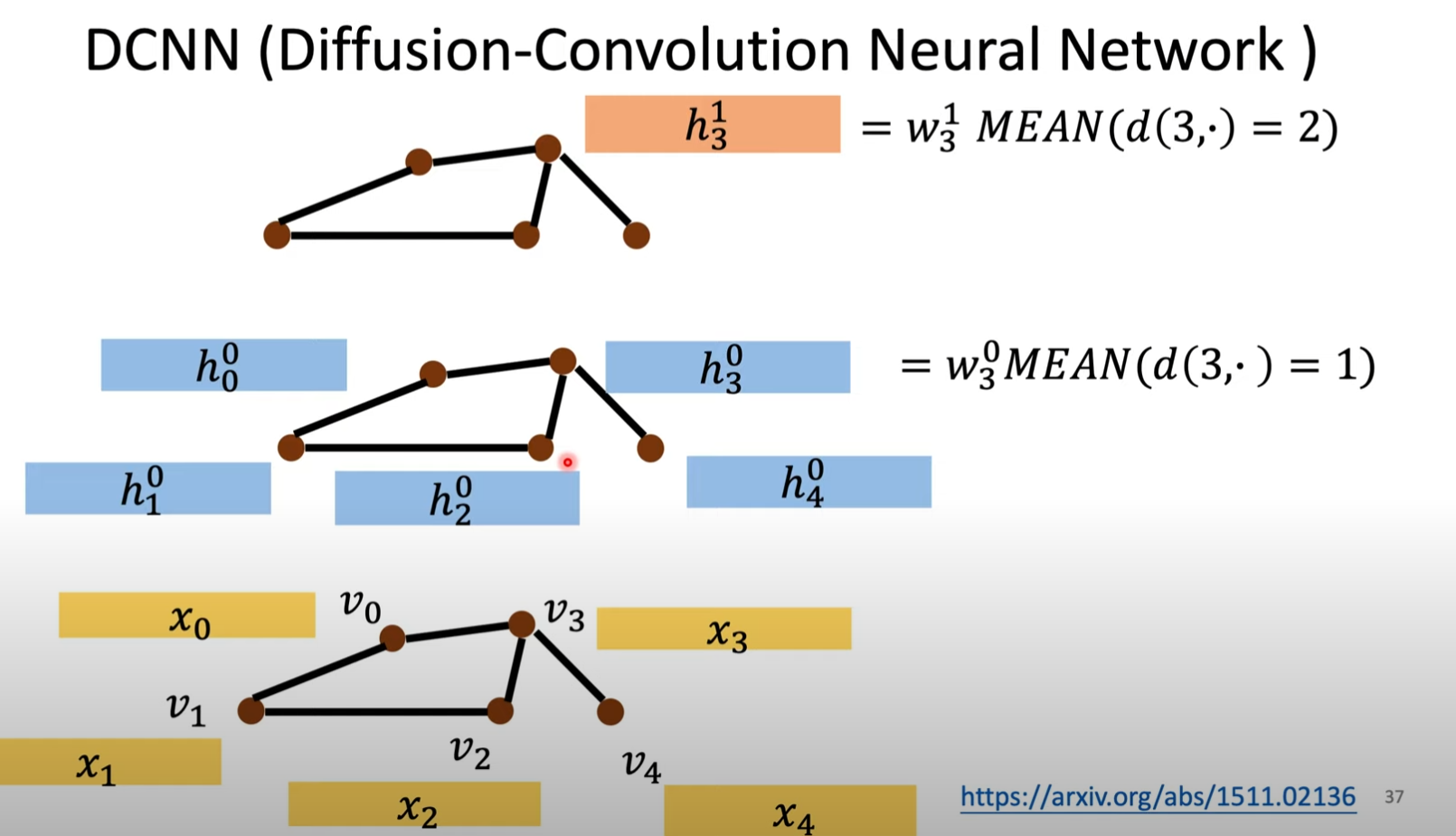$h_3^0= w_3^0 MEAN(d(3, \cdot )=1)$

$h_3^1 = w_3^1 MEAN(d(3, \cdot )=2)$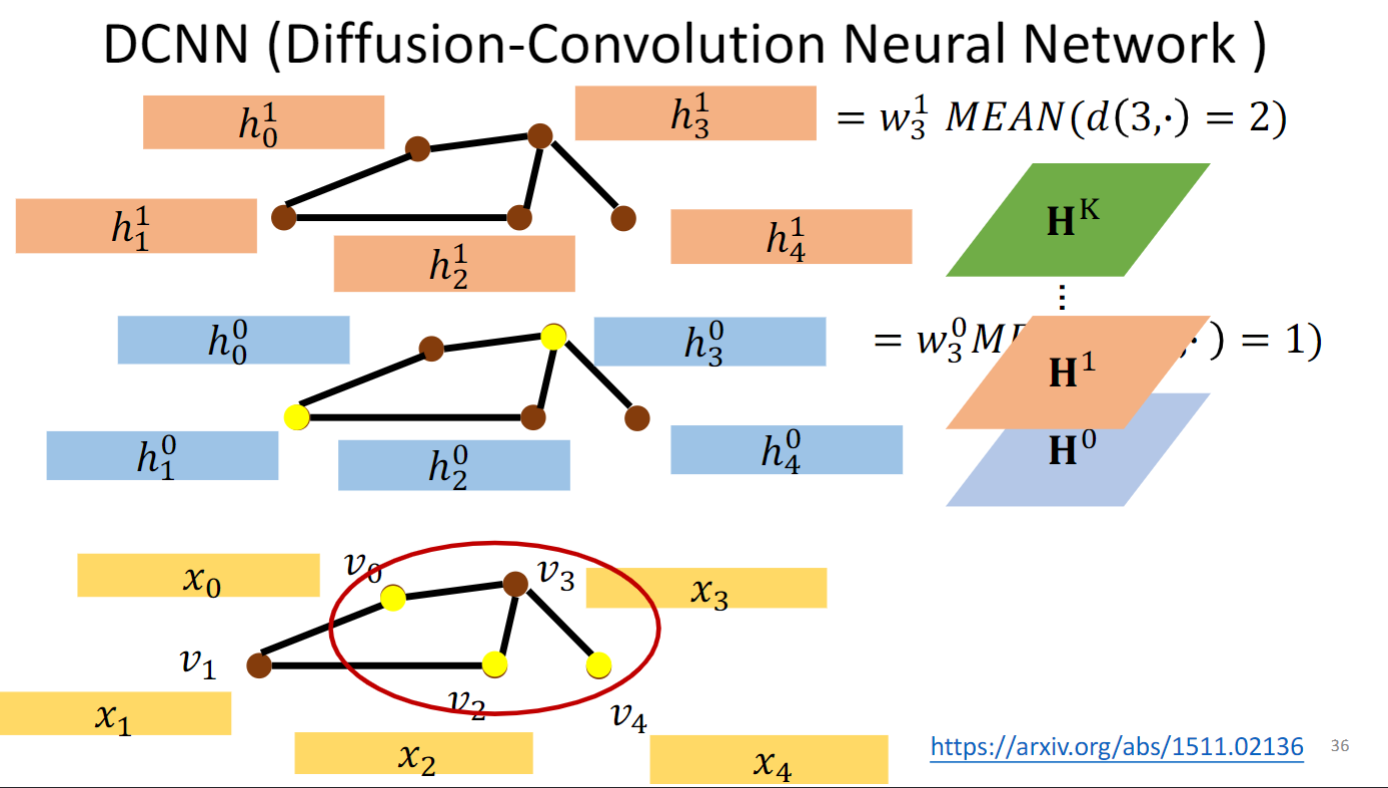$H^k$ 是将每层的h一行一行放在一起，叠成一个矩阵.### MoNET (Mixture Model Networks)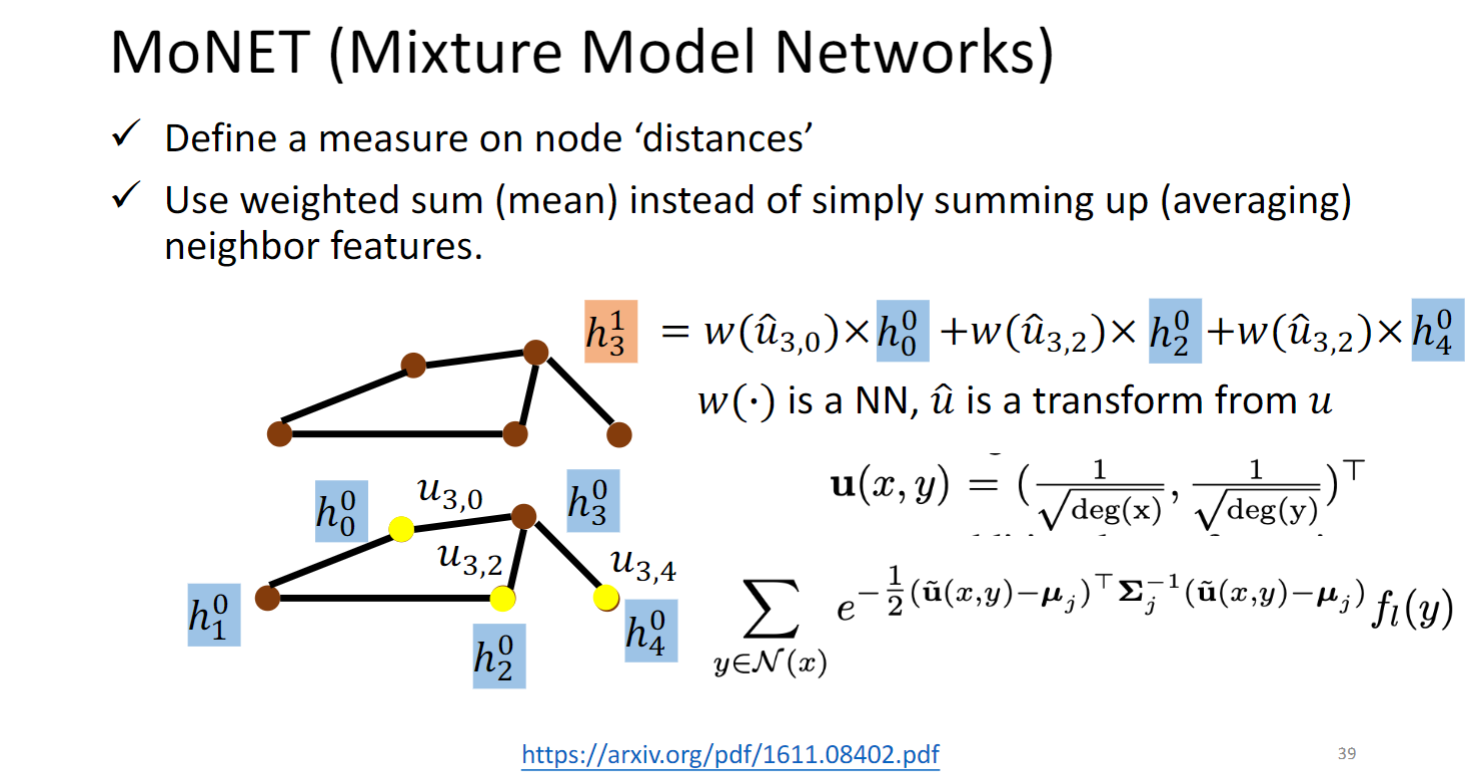MoNET 对图中的边定义了距离权重，这篇文章中距离是由公式定义的，是可以直接计算的，也有的模型（GAT ）是通过graph data学出的.

MoNET 将weighted sum neighbor features取代了NN4G和DCNN简单的相加求平均.

### GAT (Graph Attention Networks)GAT计算节点在下一层上的hidden representation的时候，先算一个节点对它所有邻接节点的energy（即可变的weight） ，然后把这个energy作为权重乘上节点在当前层上的hidden representation，再求和（如上图所示），作为最终的在下一层的表示.

### GIN (Graph Isomorphism Network)

• A GNN can be at most as powerful as WL isomorphic test
• Theoretical proofs were provided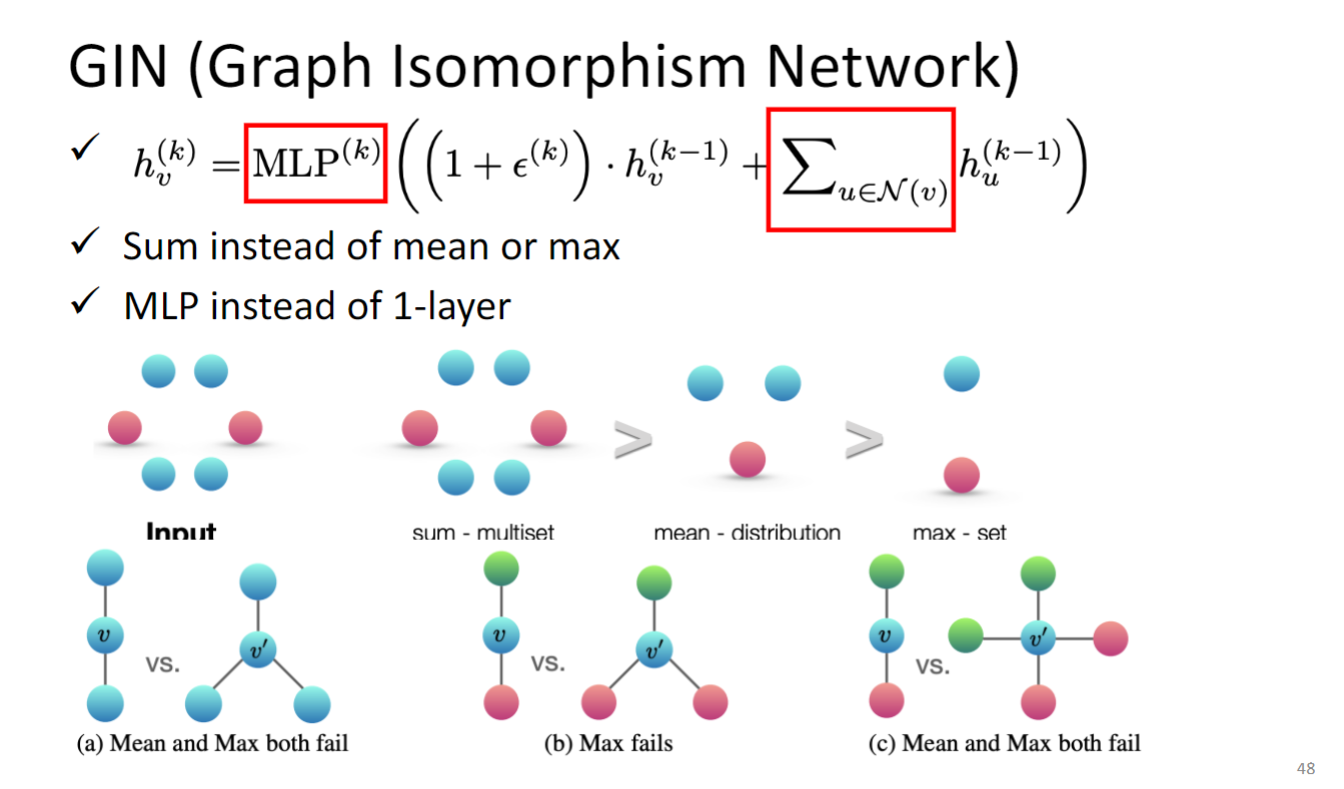$h_v^{(k)} = MLP^{(k)} ((1 + \epsilon^{(k)} )\cdot h_v^{(k-1)} + \sum_{u \in N(v)} h_v^{(k-1)})$

k是layer，v是node id，h update的方式应该要先将neighbor 全都加起来，而不能用max pooling也不能用mean pooling，然后加上某个constant 乘以 自己的feature，这个constant就是 $1 + \epsilon^{(k)}$ 这里的 $\epsilon$ 是可以学出来的，但是paper中也说了这里设为0也没太大差别.

a告诉我们，max or mean 无法区分a中的两个graph；

max无法分辨b中的两个graph；

max和mean也无法分辨c中的两个graph；

MLP是multi layer perceptron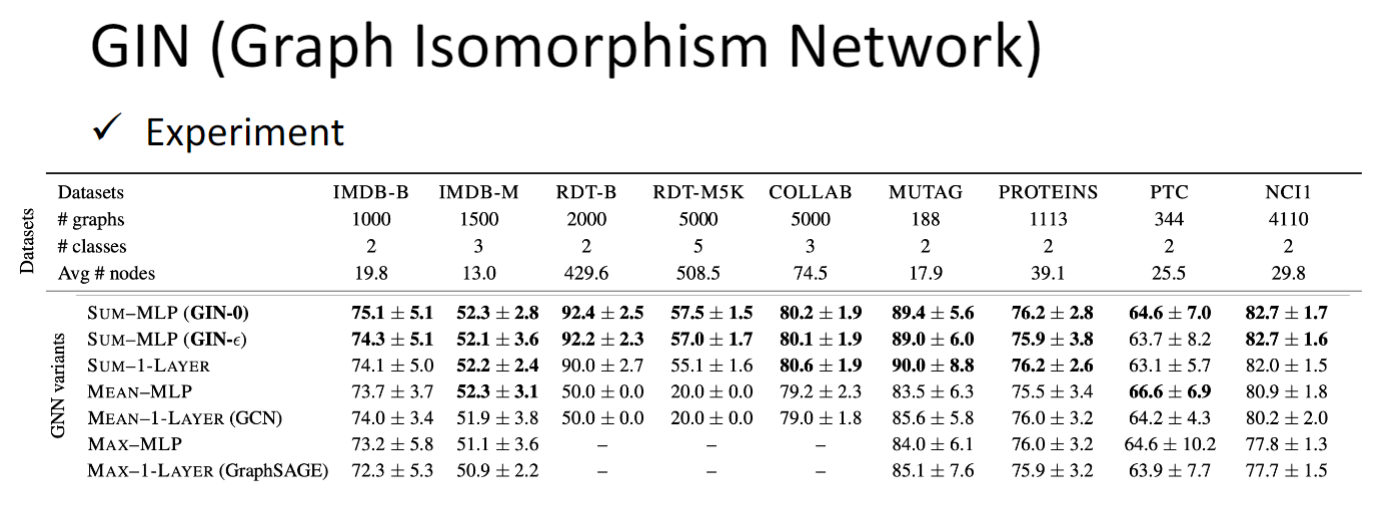## Graph Signal Processing and Spectral-based GNN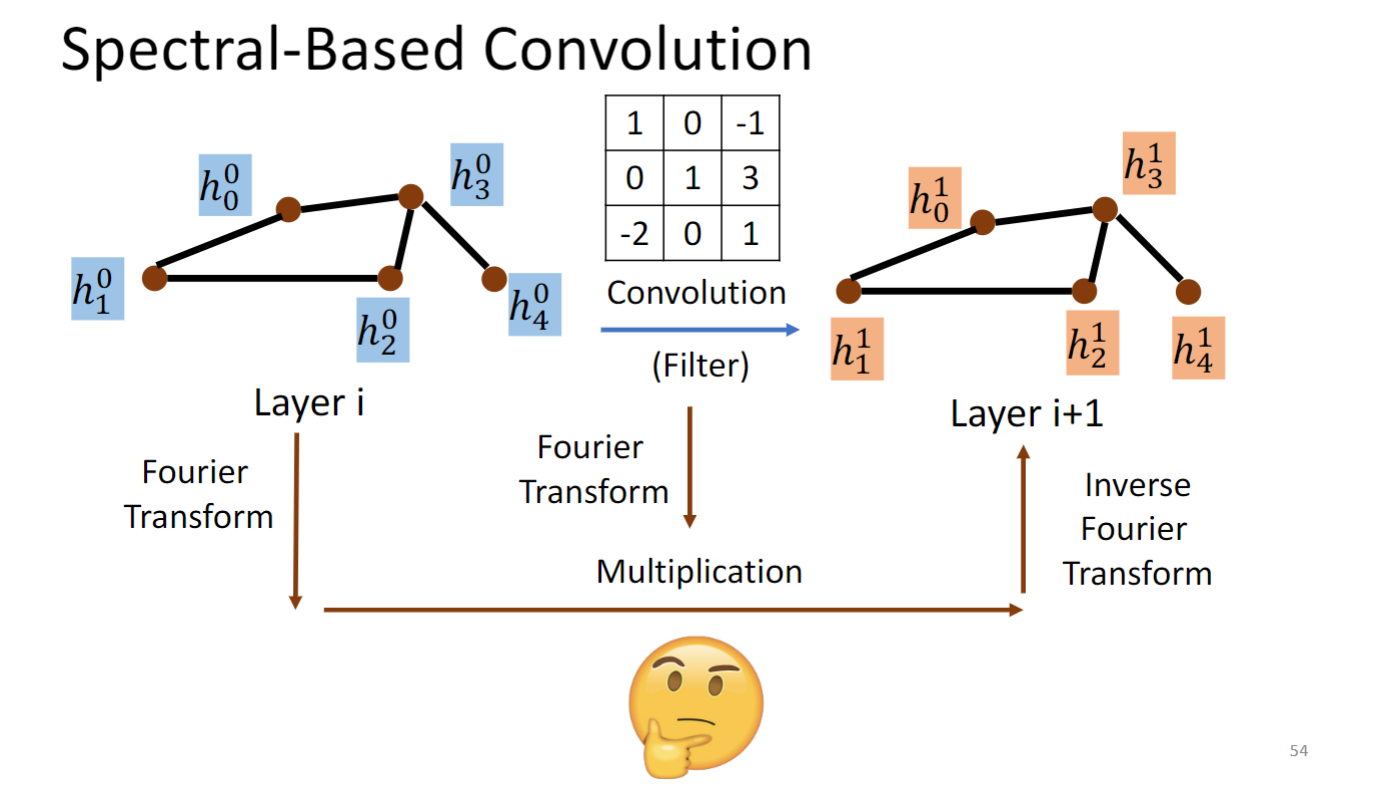Spectral-based GNN 要做的事情就是将graph 和convolution kernel 都转换到傅里叶域中，在傅里叶域中做multiplication，再转换回去，就是得到下一个layer.

### ChebNet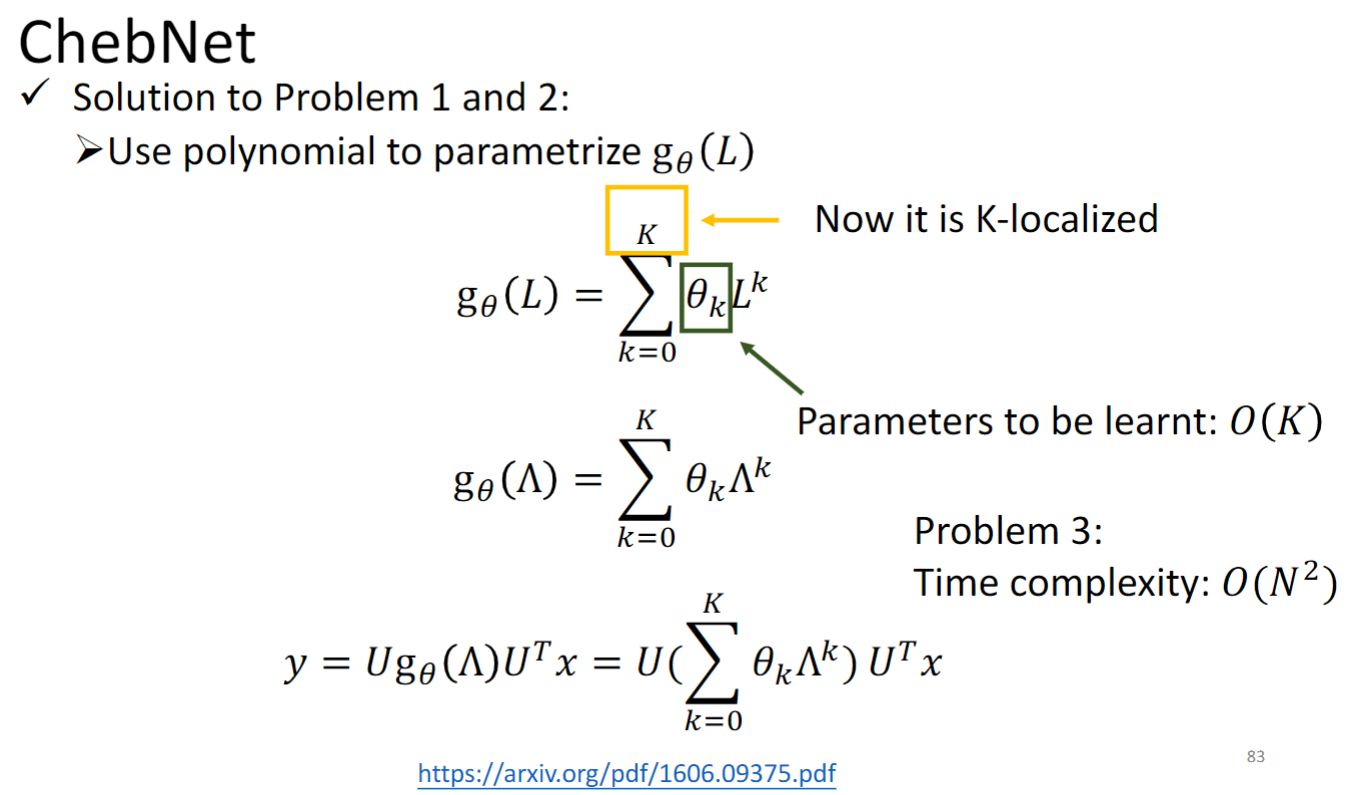$L^k$ 是拉普拉斯算子Laplacian的一个多项式函数，通过你选择让 $g_\theta(L)$ 是多项式函数的方式，你就可以让他是K-localized，因为根据上一节原理中讲的，如果你让g函数只到k次方，它就只能看到k-neighbor.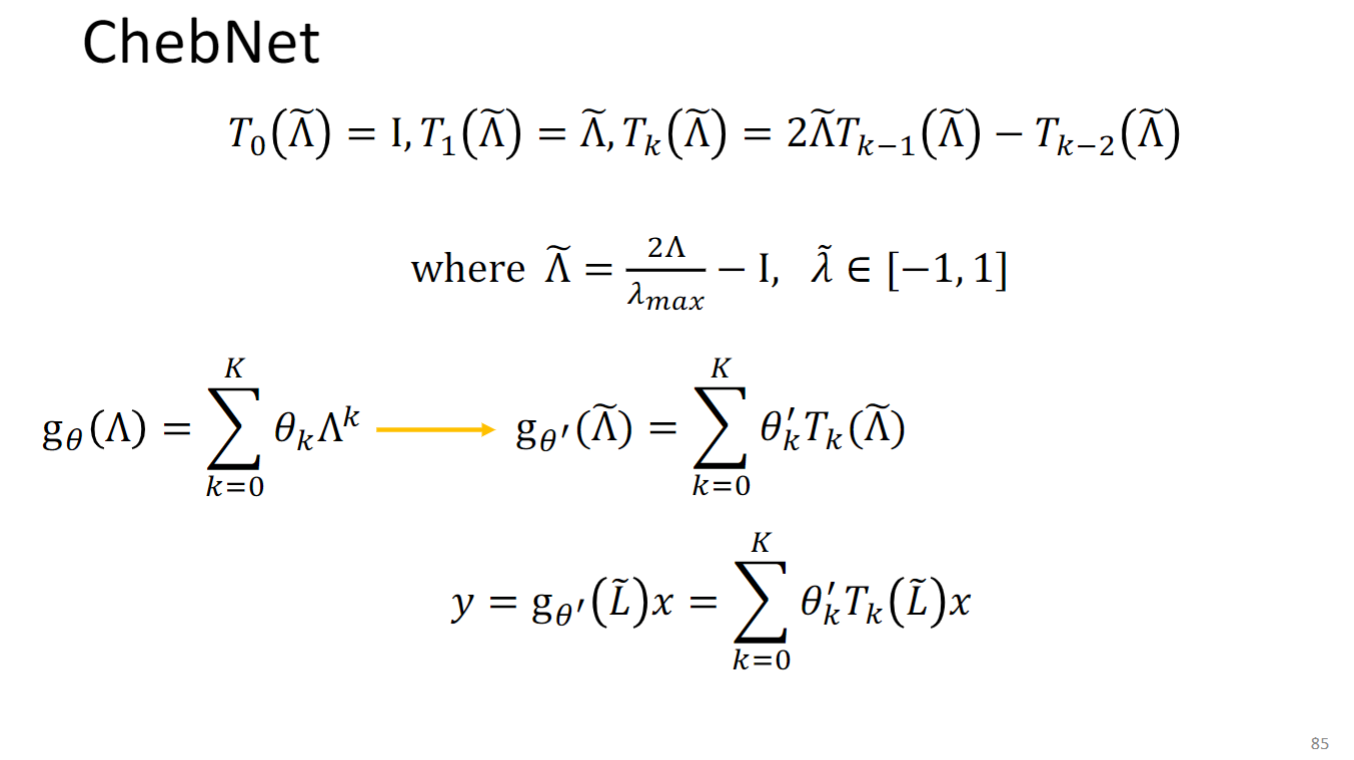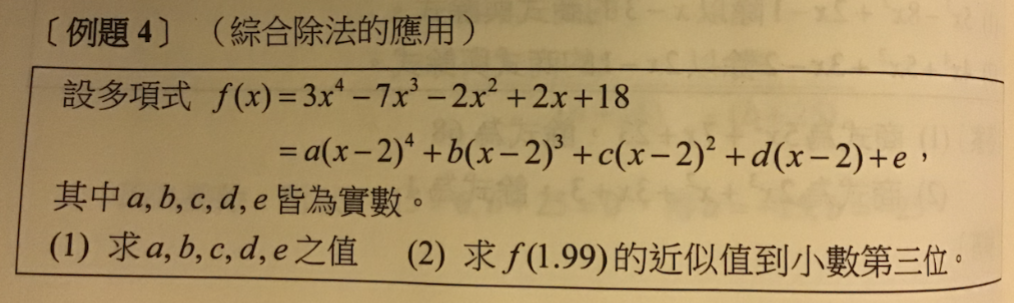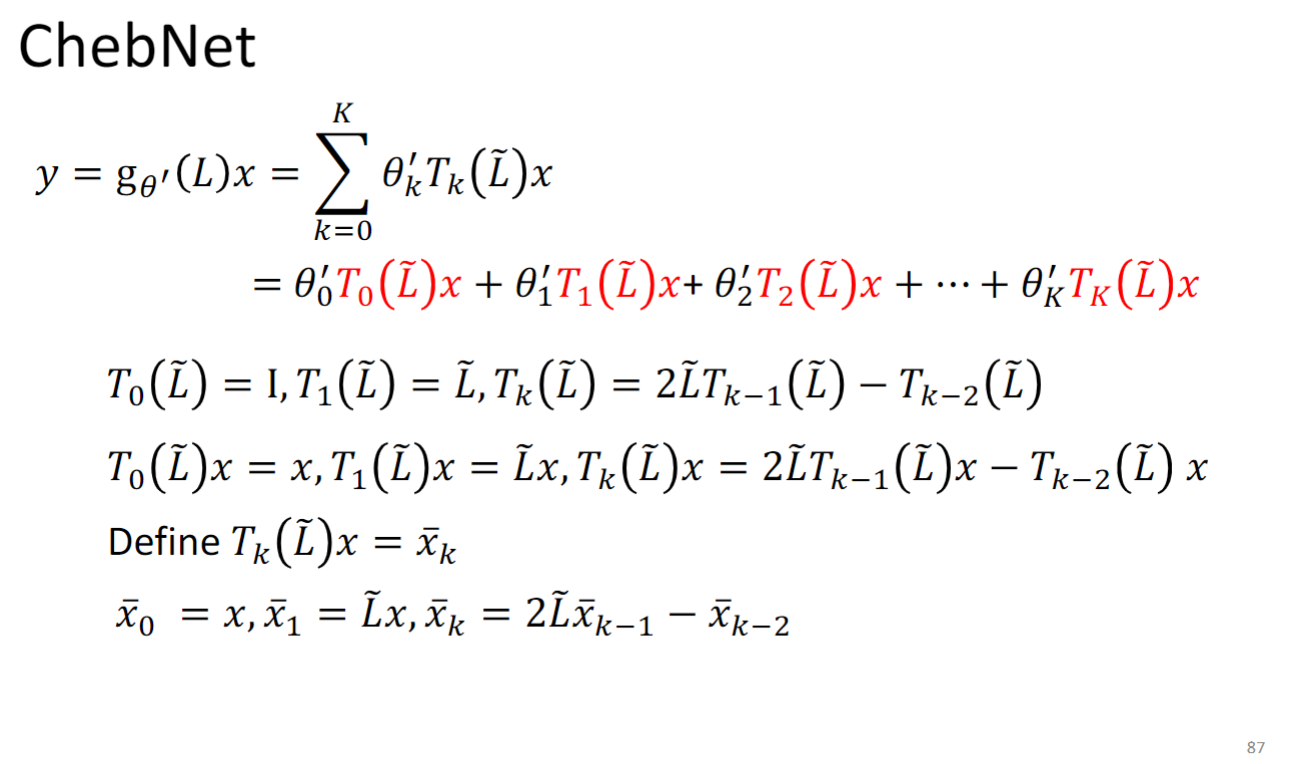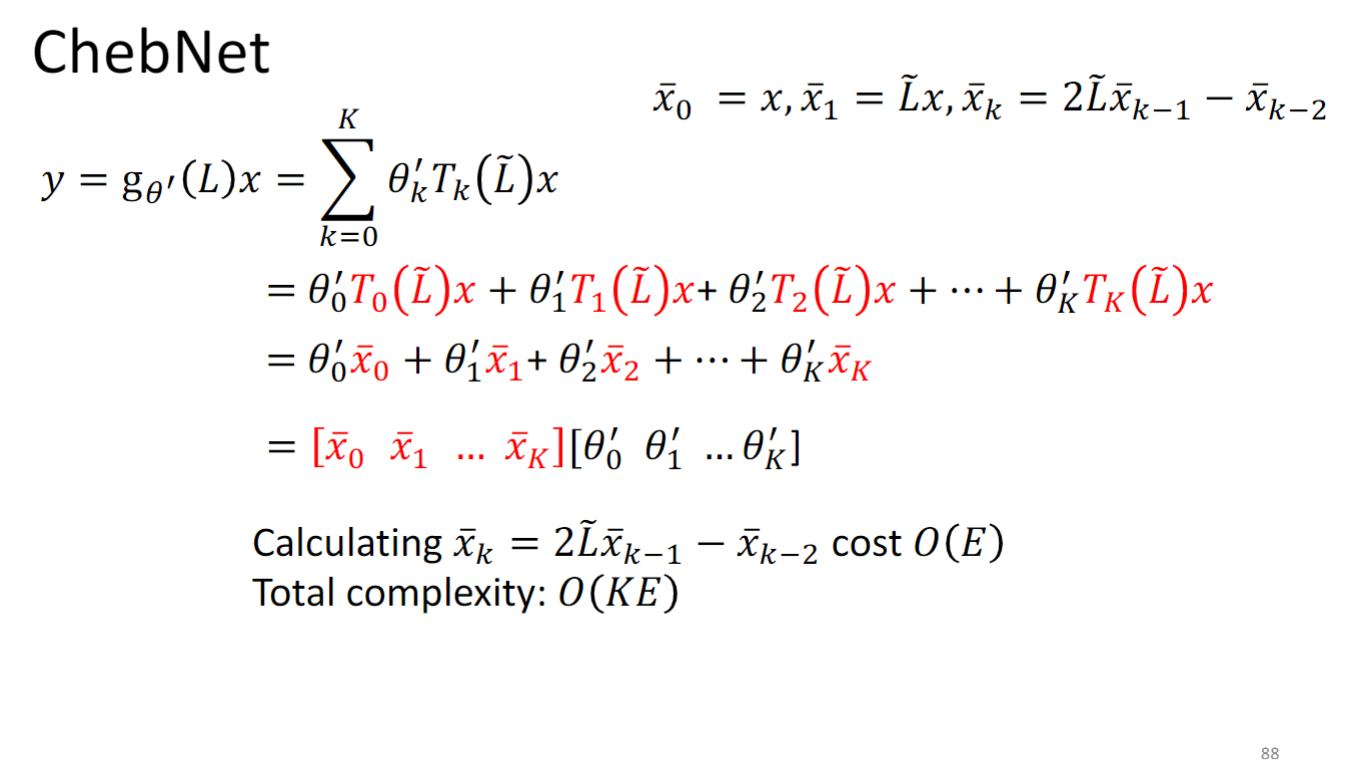### GCN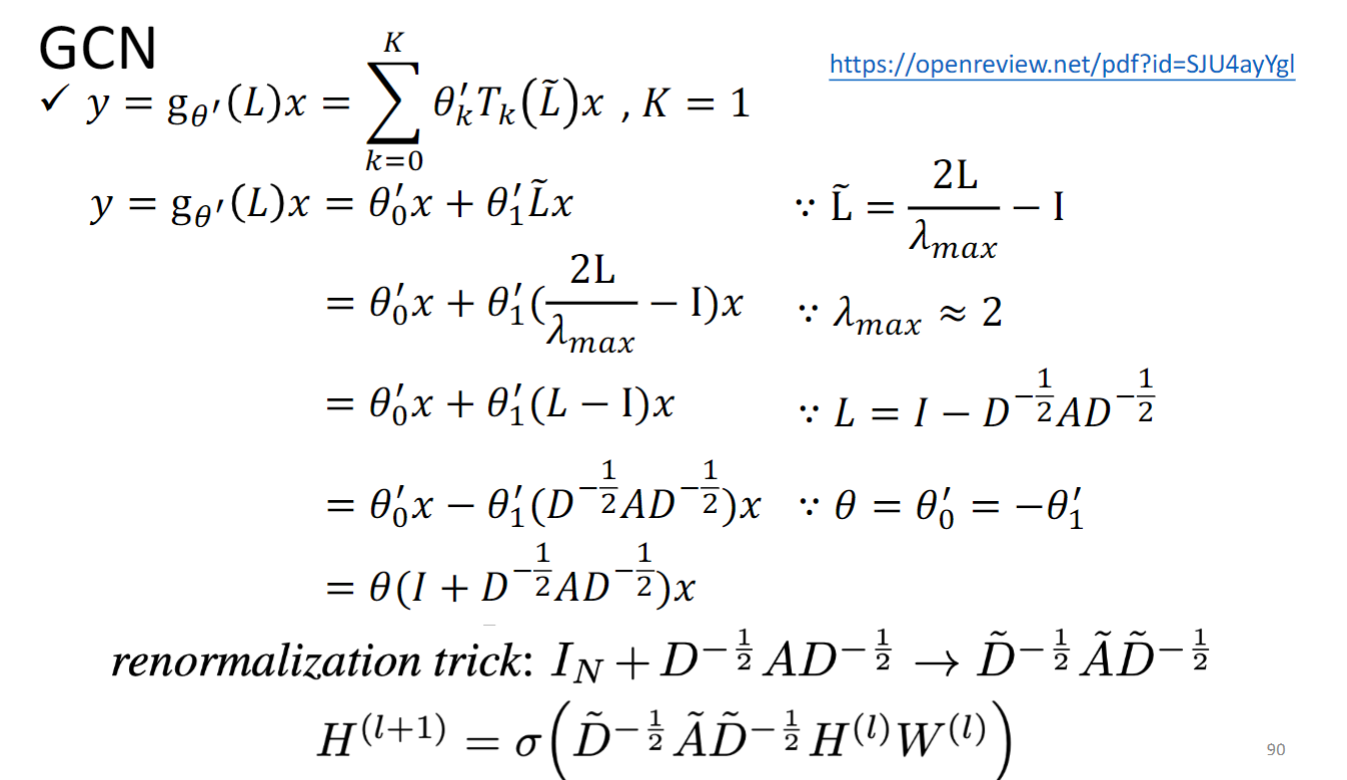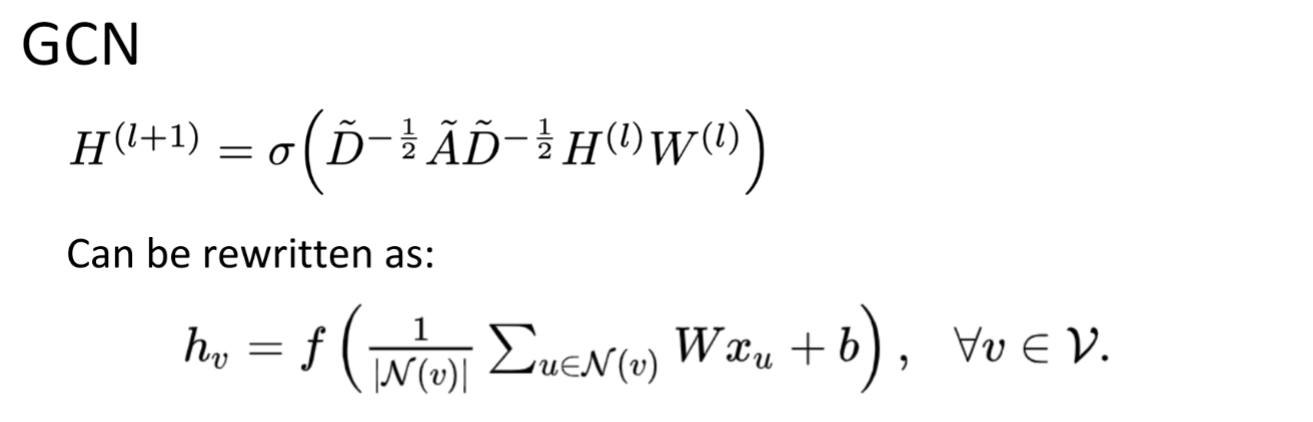GCN对每个layer中的node $h_v$ 计算就是将所有neighbor包括他自己乘一个weight，再加起来，，再加上一个bias，再经过一个nonlinear activation，就结束.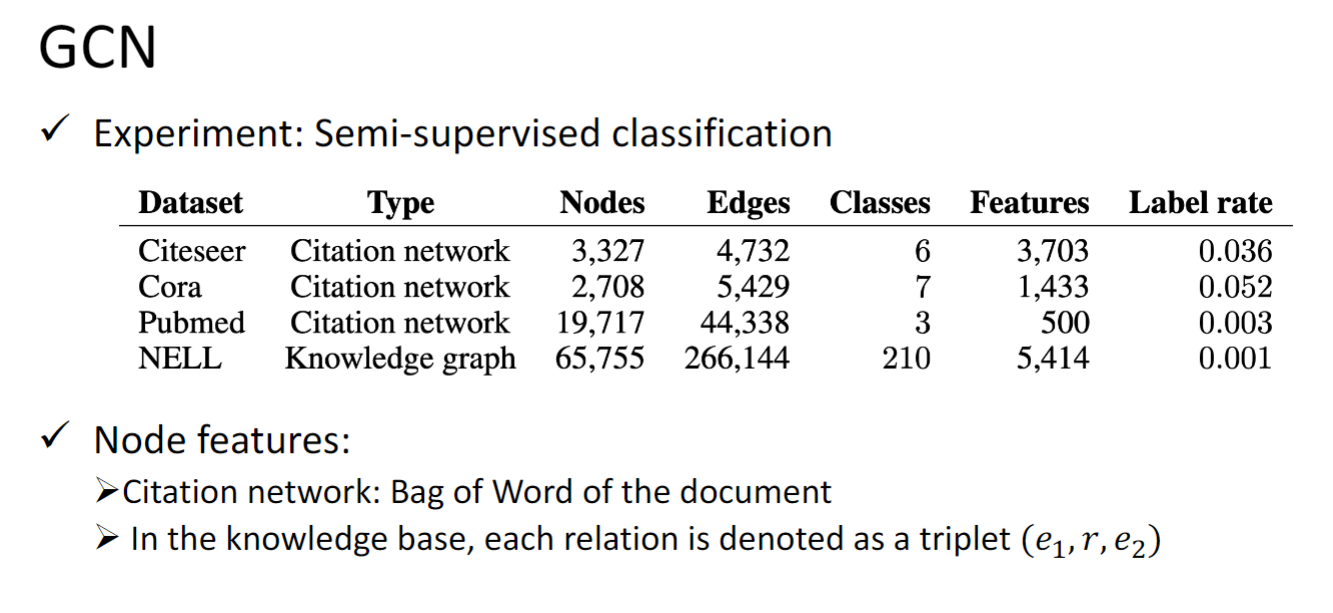## Graph Generation

• VAE-based model: Generate a whole graph in one step
• GAN-based model: Generate a whole graph in one step
• Auto-regressive- based model: Generate a node or an edge in one step

### VAE-based model